##### Managerial Economics For Dummies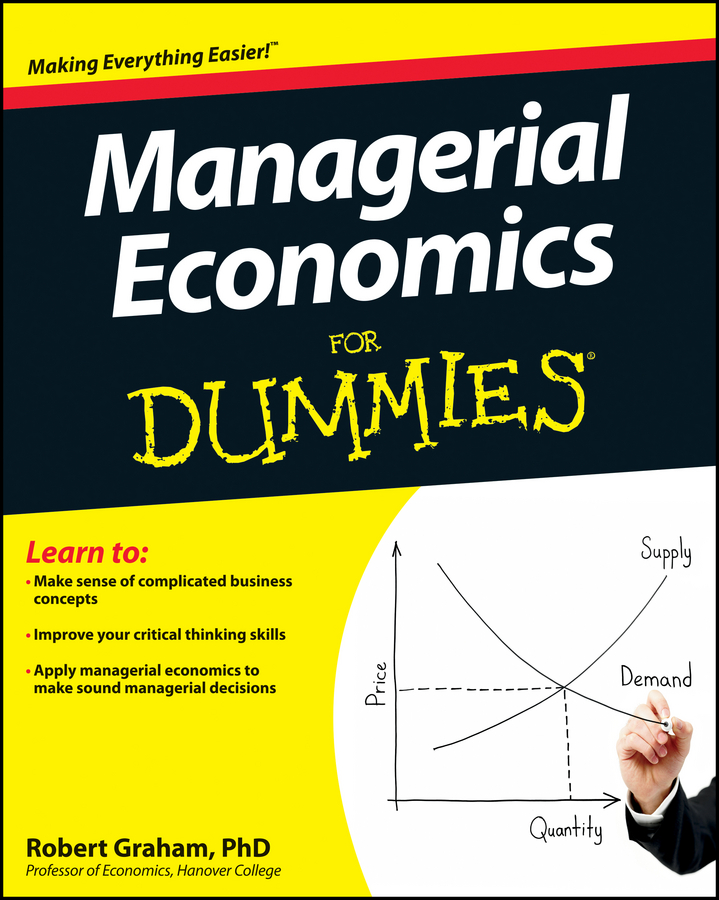There is a point where you should immediately give up and shut down your business. But first remember that going out of business in the short run doesn’t mean that your losses go to zero. Because some of the inputs you employ are fixed, going out of business in the short run means you lose your fixed costs.

Therefore, if you can make enough revenue to cover all your variable costs, you should stay in business in the short run in order to minimize your losses. However, given your goal is to maximize profits — or, in a bad situation, minimize losses — you should immediately shut down if your revenue doesn’t cover your variable costs.

Producing when your revenue is less than your variable costs means that your losses associated with the profit-maximizing quantity of output are greater than your fixed costs. You’re better off shutting down and losing only your fixed costs.

In the illustration, the profit-maximizing quantity of output, based on marginal revenue equals marginal cost, is q0. If you produce this quantity of output, your loss per unit equals price minus average total cost or the distance represented by the double-headed arrow in the illustration.

However, your fixed cost is represented by the vertical distance between average total cost, ATC0, and average variable cost, AVC0. Because this distance is less than the loss represented by the double-headed arrow, you’ll lose less money by shutting down — producing zero units of output — and limiting your losses to total fixed cost.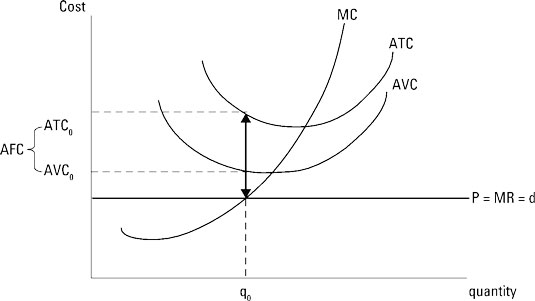Assume the market-determined price for your good is \$4.80. Therefore, your total revenue equals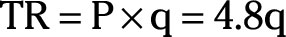Marginal revenue equals the derivative of total revenue taken with respect to quantity, or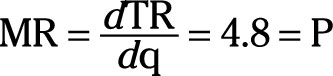If your total cost function is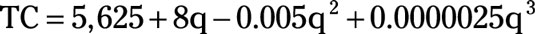Marginal cost equals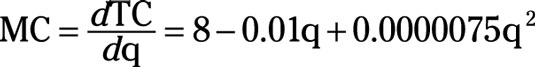Again, to determine the profit-maximizing quantity of output, set marginal revenue or price equal to marginal cost.

1. Set marginal revenue equal to marginal cost.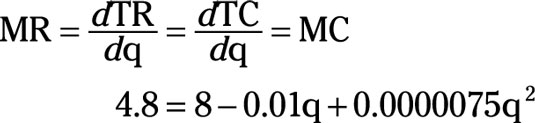2. Solve for q by using the quadratic formula.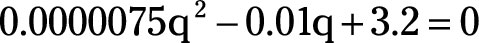The profit-maximizing quantity of output is 800 units.

3. Determine the average-total-cost equation.

Average total cost equals total cost divided by the quantity of output.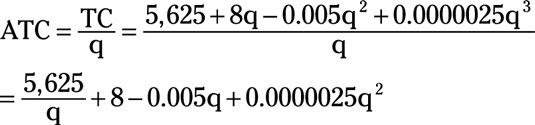4. Substitute the profit-maximizing quantity of 800 for q to determine average total cost.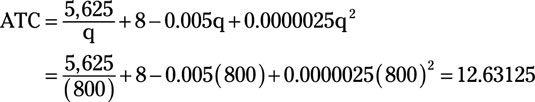5. Calculate profit per unit.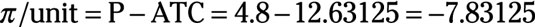or profit per unit equals –\$7.83125.

6. Determine total profit by multiplying profit per unit by the profit-maximizing quantity of output.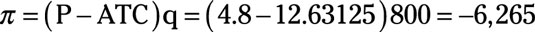or total profit equals –\$6,265. By producing 800 units of output where marginal revenue equals marginal cost, your firm is losing \$6,265. But note that if you immediately shut down, your losses equal total fixed cost, which is only \$5,625. Losing \$5,625 is a bad situation, but losing \$6,265 is even worse. Shutting down immediately becomes your best option for minimizing your losses.